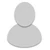## FANDOM

5,869 Pages

• First pic: max rime rounds + max cryo rounds = +150% damage.

62.5 = 2.5x of 25. correct.

Second pic: rank 9 serration = +150% damage, too... or is it?

62.0 = 2.48x of 25... wut?

• I don't get the problem, is the ",2" with serration? it must be that with two mods it round the amount downward

• Base damage for Paracyst is 25. The first pic shows that +60% damage from Rime Rounds and +90% damage from Cryo Rounds, equal to +150% damage, increases Paracyst damage to 62.5, which is correct. (25*1 + 25*0.6 + 25*0.9 or 25*1 + 25*1.5)

But the second pic shows that +150% damage from serration only increases damage to 62.0, which is 25*1 + 25*1.48 instead of 1.5.

•